Courses

# Analytical Reasoning MCQ Quiz - 1

## 10 Questions MCQ Test Quantitative Aptitude for Banking Preparation | Analytical Reasoning MCQ Quiz - 1

Description
This mock test of Analytical Reasoning MCQ Quiz - 1 for UPSC helps you for every UPSC entrance exam. This contains 10 Multiple Choice Questions for UPSC Analytical Reasoning MCQ Quiz - 1 (mcq) to study with solutions a complete question bank. The solved questions answers in this Analytical Reasoning MCQ Quiz - 1 quiz give you a good mix of easy questions and tough questions. UPSC students definitely take this Analytical Reasoning MCQ Quiz - 1 exercise for a better result in the exam. You can find other Analytical Reasoning MCQ Quiz - 1 extra questions, long questions & short questions for UPSC on EduRev as well by searching above.
QUESTION: 1

### Find the number of triangles in the given figure.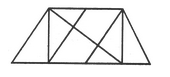Solution:

The figure may be labelled as shown.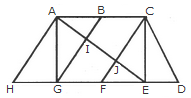The simplest triangles are AHG, AIG, AIB, JFE, CJE and CED i.e. 6 in number.

The triangles composed of two components each are ABG, CFE, ACJ and EGI i.e. 4 in number.

The triangles composed of three components each are ACE, AGE and CFD i.e. 3 in number.

There is only one triangle i.e. AHE composed of four components.

Therefore, There are 6 + 4 + 3 + 1 = 14 triangles in the given figure.

QUESTION: 2

### Find the number of triangles in the given figure.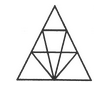Solution:

The figure may be labelled as shown.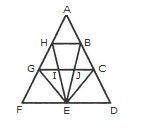The simplest triangles are AHB, GHI, BJC, GFE, GIE, IJE, CEJ and CDE i.e. 8 in number.

The triangles composed of two components each are HEG, BEC, HBE, JGE and ICE i.e. 5 in number.

The triangles composed of three components each are FHE, GCE and BED i.e. 3 in number.

There is only one triangle i.e. AGC composed of four components.

There is only one triangle i.e. AFD composed of nine components.

Thus, there are 8 + 5 + 3 + 1 + 1 = 18 triangles in the given figure.

QUESTION: 3

### Find the number of triangles in the given figure.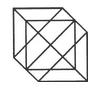Solution:

The figure may be labelled as shown.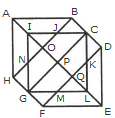The simplest triangles are IJO, BCJ, CDK, KQL, MLQ, GFM, GHN and NIO i.e. 8 in number.

The triangles composed of two components each are ABO, AHO, NIJ, IGP, ICP, DEQ, FEQ, KLM, LCP and LGP i.e.10 in number.

The triangles composed of four components each are HAB, DEF, LGI, GIC, ICL and GLC i.e. 6 in number.

Total number of triangles in the figure = 8 + 10 + 6 = 24.

QUESTION: 4

Find the number of triangles in the given figure.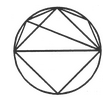Solution:

The figure may be labelled as shown.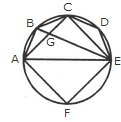The simplest triangles are ABG, BCG, CGE, CDE, AGE and AEF i.e. 6 in number.

The triangles composed of two components each are ABE, ABC, BCE and ACE i.e. 4 in number.

There are 6 + 4 = 10 triangles in the figure.

QUESTION: 5

Find the number of triangles in the given figure.Solution:

The figure may be labelled as shown.The simplest triangles are BFG, CGH, EFM, FMG, GMN, GHN, HNI, LMK, MNK and KNJ i.e. 10 in number.

The triangles composed of three components each are FAK and HKD i.e. 2 in number.

The triangles composed of four components each are BEN, CMI, GLJ and FHK i.e. 4 in number.

The triangles composed of eight components each are BAJ and OLD i.e. 2 in number.

Thus, there are 10 + 2 + 4 + 2 = 18 triangles in the given figure.

QUESTION: 6

Count the number of rectangles in the given figure.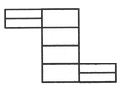Solution:

The figure may be labelled as shown.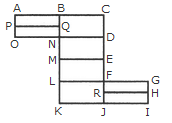The simplest rectangles are ABQP, PQNO, BCDN, NDEM, MEFL, LFJK, FGHR and RHIJ i.e. 8 in number.

The rectangles composed of two components each are ABNO, BCEM, NDFL, MEJK and FGIJ i.e. 5 in number.

The rectangles composed of three components each are ACDO, BCFL, NDJK and LGIK i.e. 4 in number.

There is only one rectangle i.e. BCJK composed of four components.

Total number of rectangles in the figure = 8 + 5 + 4 + 1 = 18.

QUESTION: 7

How many circles are there in the adjoining figure.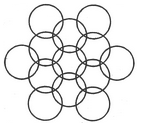Solution:

The figure may be labelled as shown.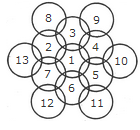There are 13 circles in the given figure. This is clear from the adjoining figure in which the centres of all the circles in the given figure have been numbered from 1 to 13.

QUESTION: 8

What is the minimum number of straight lines that is needed to construct the figure?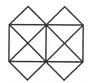Solution:

The figure may be labelled as shown.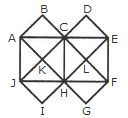The horizontal lines are AE and JF i.e. 2 in number. The vertical lines are AJ, CH and EF i.e. 3 in number.

The slanting lines are AG, BF, JD, IE, AB, DE, JI and FG i.e. 8 in number.

Total number of straight lines needed to construct the figure = 2 + 3 + 8 = 13.

QUESTION: 9

Determine the number of rectangles and hexagons in the given figure.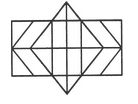Solution:

The figure may be labelled as shown.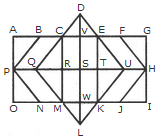Rectangles:

The simplest rectangles are CVSR, VETS, RSWM and STKW i.e. 4 in number.

The rectangles composed of two components each are CETR, VEKW, RTKM and CVWM i.e. 4 in number.

The rectangles composed of three components each are ACRP, PRMO, EGHT and THIK i.e. 4 in number.

The rectangles composed of four components each are CEKM, AVSP,PSWO, VGHS and SHIW i.e. 5 in number.

The rectangles composed of five components each are AETP, PTKO, CGHR and RHIM i.e. 4 in number.

The rectangles composed of six components each are ACMO and EGIK i.e. 2 in number.

The rectangles composed of eight components each are AGHP, PHIO, AVWO and VGIW i.e. 4 in number.

The rectangles composed of ten components each are AEKO and CGIM i.e. 2 in number.

AGIO is the only rectangle having sixteen components.

Total number of rectangles in the given figure

= 4 + 4 + 4 + 5 + 4 + 2 + 4 + 2 + 1 = 30.

Hexagons:

The hexagons in the given figure are CDEKLM, CEUKMQ, CFHJMQ, BEUKNP and BFHJNP.

So, there are 5 hexagons in the given figure.

QUESTION: 10

Count the number of triangles and squares in the given figure.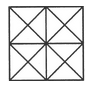Solution:

The figure may be labelled as shown.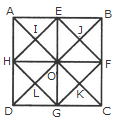Triangles :

The simplest triangles are AEI, EOI, OHI, HAI, EBJ, BFJ, FOJ, OEJ, HOL, OGL, GDL, DHL, OFK, FCK, CGK and GOK i.e. 16 in number.

The triangles composed of two components each are HAE, AEO, EOH, OHA, OEB, EBF, BFO, FOE, DHO, HOG, OGD, GDH, GOF, OFC, FCG and CGO i.e. 16 in number.

The triangles composed of four components each are HEF, EFG, FGH, GHE, ABO, BGO, CDO and DAO i.e. 8 in number.

The triangles composed of eight components each are DAB, ABC, BCD and CDA i.e. 4 in number.

Total number of triangles in the figure = 16 + 16 + 8 + 4 = 44.

Squares :

The squares composed of two components are HIOL, IEJO, JFKO and KGLO i.e. 4 in number.

The squares composed of four components are AEOH, EBFO, OFGC and HOGD i.e.4 in number.

There is only one square EFGH which is composed of eight components.

There is only one square ABCD which is composed of sixteen components.

Total number of squares in the figure = 4 + 4 + 1 + 1 = 10.

Track your progress, build streaks, highlight & save important lessons and more!

### Similar Content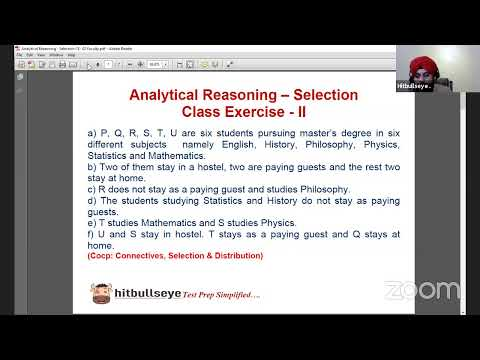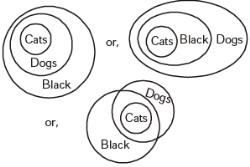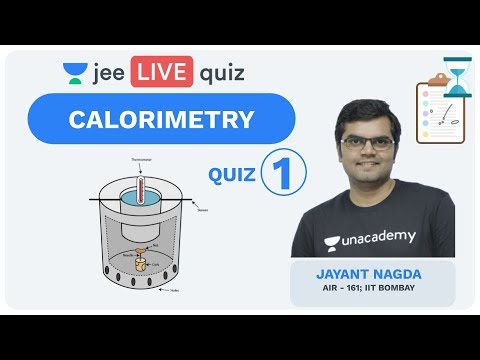### Related tests